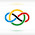### 2017 IMO SL #N6

Find the smallest positive integer $n$ or show no such $n$ exists, with the following property: there are infinitely many distinct $n$-tuples of positive rational numbers $(a_1, a_2, \ldots, a_n)$ such that both $$a_1+a_2+\dots +a_n \quad \text{and} \quad \frac{1}{a_1} + \frac{1}{a_2} + \dots + \frac{1}{a_n}$$are integers.

The answer is $n=3$.

We will show that that $n=1,2$ don't work and $n=3$ does.

Clearly, $n=1$ does not work, as there are only finitely many such tuples, namely $1$ and $-1$.

For the $n=2$ case, consider rationals $a_1=\frac{a}{b}, a_2=\frac{c}{d}$ where $\gcd(a,b)=\gcd(c,d)=1$. Note that $$\frac{a}{b}+\frac{c}{d}=\frac{ad+bc}{bd}\in\mathbb{Z}$$therefore $b\mid ad+bc\implies b\mid ad\implies b\mid d$. Similarly $d\mid b$ hence $b=d$. Since $\frac{b}{a}+\frac{d}{c}\in\mathbb{Z}$, repeating similar logic gives $a=c$. These two together give $a_1=a_2$, so these two rationals are not distinct, and hence $n=2$ doesn't work.

Now to show $n=3$ works, consider $(a_1, a_2, a_3) = \left(\frac1{m+n+1},\frac m{m+n+1},\frac n{m+n+1}\right)$ for positive integers $1<m<n$. The $a_i$ are distinct, and $a_1 + a_2 + a_3 = 1$, so $\frac{m+1}n+\frac{n+1}m$ must be a positive integer for infinitely many pairs $(m,n)$ for $1<m<n$.

Write this as$m^2 + n^2 + m + n = mnk$for some positive integer $k$. As a quadratic in $m$, this becomes$m^2 + (1 - kn)m + (n^2 + n) = 0$so by Vieta's, given the solution $(m, n)$ with $m < n$, it then follows that$(kn - 1 - n, n) \longleftrightarrow (n, kn - 1 - m)$is also a solution. Note $kn - 1 - m > n$ for all $k > 2$. Therefore, if we are given any initial solution $(m_0, n_0)$ and some fixed $k$, we can compute infinitely many solutions by $(m_{i+1}, n_{i+1}) = (n_i, kn_i - 1 - m_i)$ where $\min(m_i, n_i)$ is increasing. Note that for $k = 3$ there exists an initial solution $(m_0, n_0) = (2, 3)$, so using the method defined above, we can produce as many $(m_i, n_i)$ we want, as desired. $\square$

1.Anonymous7/04/2022

very informati

1.Thank you!